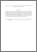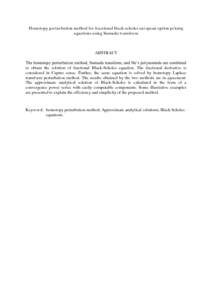Simple Search:

Homotopy perturbation method for fractional black-scholes european option pricing equations using Sumudu transform

Citation

Elbeleze, Asma Ali and Kilicman, Adem and M. Taib, Bachok (2013) Homotopy perturbation method for fractional black-scholes european option pricing equations using Sumudu transform. Mathematical Problems in Engineering, 2013. art. no. 524852. pp. 1-7. ISSN 1024-123X

Abstract / Synopsis

The homotopy perturbation method, Sumudu transform, and He’s polynomials are combined to obtain the solution of fractional Black-Scholes equation. The fractional derivative is considered in Caputo sense. Further, the same equation is solved by homotopy Laplace transform perturbation method. The results obtained by the two methods are in agreement. The approximate analytical solution of Black-Scholes is calculated in the form of a convergence power series with easily computable components. Some illustrative examples are presented to explain the efficiency and simplicity of the proposed method.Preview
PDF (Abstract)
Homotopy perturbation method for fractional black.pdfView Item